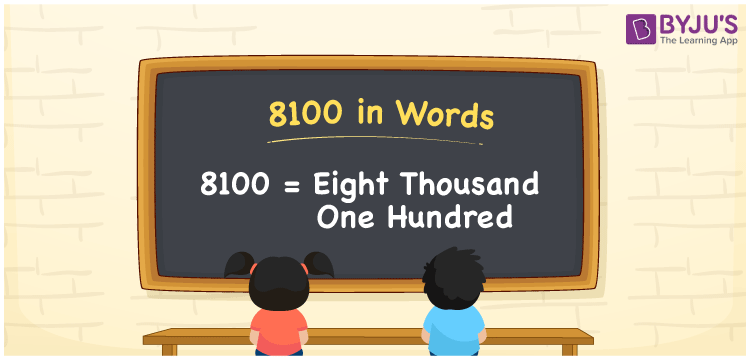# 8100 in Words

8100 in words is written as Eight thousand one hundred. In both the International System of Numerals and the Indian System of Numerals, 8100 is written as Eight thousand one hundred. The number 8100 is a Cardinal Number as it represents some quantity, “how many of something” or “how much of something”. For example, the dress costs 8100 rupees.

 8100 in words Eight thousand one hundred Eight thousand one hundred in Number 8100

## 8100 in English Words

8100 in English words is read as “Eight thousand one hundred.”## How to Write 8100 in Words?

To write 8100 in words, we shall use the place value chart. In the place value chart, put 8 in the thousands, 1 in the hundreds, and 0 in the rest. Let us make a place value chart to write the number 8100 in words.

 Thousands Hundreds Tens Ones 8 1 0 0

Thus, we can write the expanded form as

8 × Thousand + 1 × Hundred + 0 × Ten + 0 × One

= 8 × 1000 + 1 × 100 + 0 × 10 + 0 × 1

= 8000 + 100 + 0 + 0

= 8100

= Eight thousand one hundred.

8100 is the natural number that is succeeded by 8099 and preceded by 8101.

8100 in words – Eight thousand one hundred

• Is 8100 an odd number? – No
• Is 8100 an even number? – Yes
• Is 8100 a perfect square number? – Yes; 90 × 90 = 8100
• Is 8100 a perfect cube number? – No
• Is 8100 a prime number? – No
• Is 8100 a composite number? – Yes

## Frequently Asked Questions on 8100 in Words

Q1

### How to write 8100 in words?

8100 in words is written as Eight thousand one hundred.
Q2

### How to write 8100 in words in the International and Indian System of Numerals?

In both, the system of numerals, 8100 in words, is written as Eight thousand one hundred.
Q3

### How to write 8100 in a place value chart?

In the place value chart, write 8 in the thousands, 1 in the hundreds and 0 in the tens and the ones, respectively.